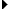Android APIs
public interface

# TypeEvaluator

 android.animation.TypeEvaluatorKnown Indirect Subclasses

## Class Overview

Interface for use with the `setEvaluator(TypeEvaluator)` function. Evaluators allow developers to create animations on arbitrary property types, by allowing them to supply custom evaluators for types that are not automatically understood and used by the animation system.

• `setEvaluator(TypeEvaluator)`

## Summary

Public Methods
abstract T evaluate(float fraction, T startValue, T endValue)
This function returns the result of linearly interpolating the start and end values, with `fraction` representing the proportion between the start and end values.

## Public Methods

#### public abstract T evaluate(float fraction, T startValue, T endValue)

This function returns the result of linearly interpolating the start and end values, with `fraction` representing the proportion between the start and end values. The calculation is a simple parametric calculation: `result = x0 + t * (x1 - x0)`, where `x0` is `startValue`, `x1` is `endValue`, and `t` is `fraction`.
• A linear interpolation between the start and end values, given the `fraction` parameter.